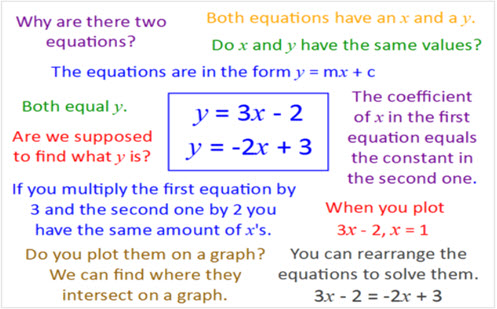Go to website

# Intersecting lines

The lesson aims to challenge students through exploring their understanding of linear equations and intersecting lines. Students work collaboratively, following guided questions to explore the relationships between two linear equations. The lesson is aimed to challenge students' thinking, encouraging them to explore and deepen their conceptual understanding. The resource includes classroom examples, teacher notes and guided questions.

Year level(s) Year 10
Audience Teacher
Teaching strategies and pedagogical approaches Collaborative learning, Feedback, Mathematics investigation
Keywords linear equations, iIntersecting lines, graph, gradient, y-intercept

## Curriculum alignment

Curriculum connections Critical and creative thinking, Numeracy
Strand and focus Space, Algebra, Apply understanding
Topics Algebraic expressions, Linear relationships
AC: Mathematics (V9.0) content descriptions
AC9M10A02

Solve linear inequalities and simultaneous linear equations in 2 variables; interpret solutions graphically and communicate solutions in terms of the situation

Numeracy progression Number patterns and algebraic thinking (P9)